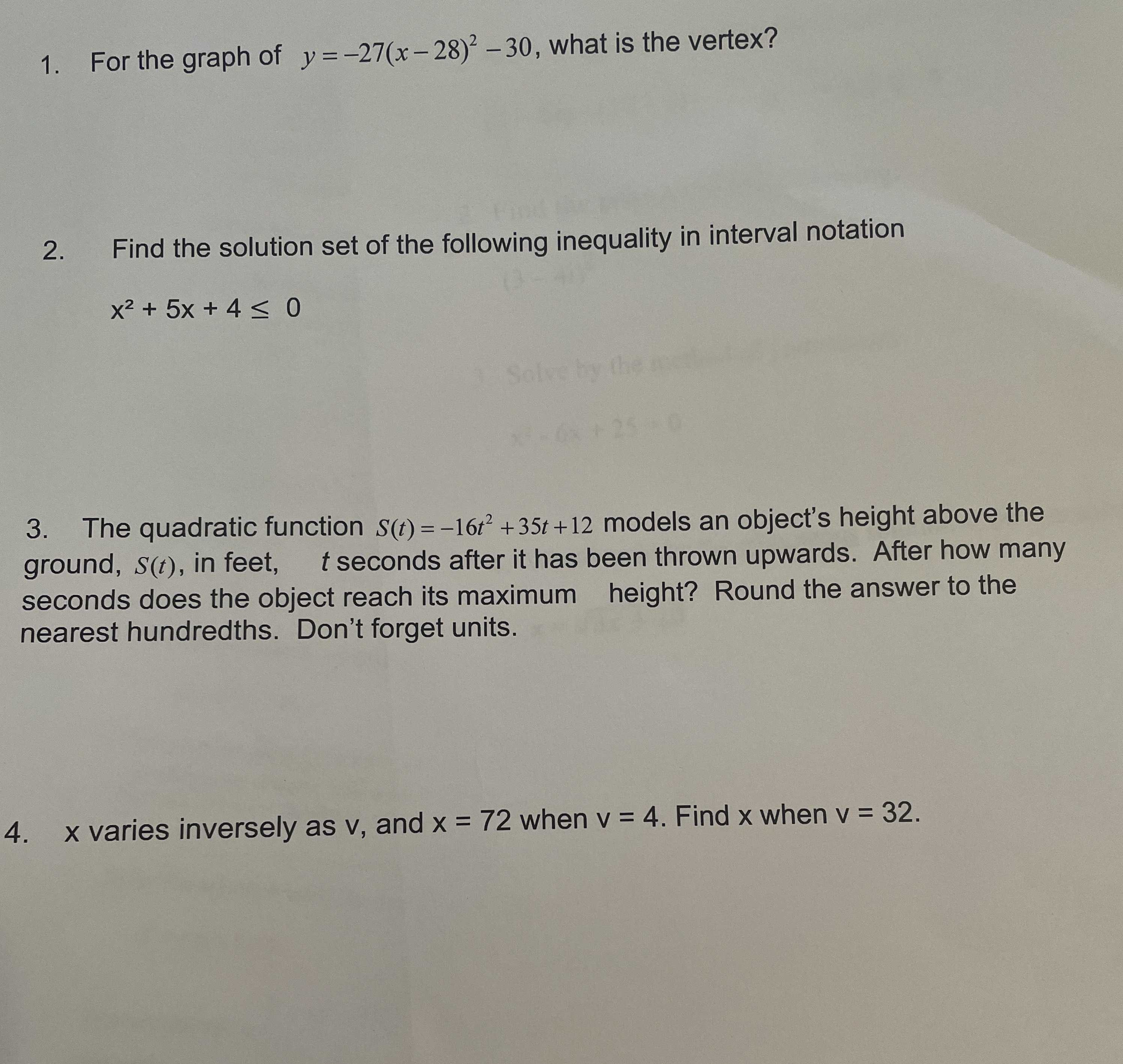### ¿Todavía tienes preguntas de matemáticas?

Pregunte a nuestros tutores expertos
Algebra
Pregunta1. For the graph of $$y = - 27 ( x - 28 ) ^ { 2 } - 30$$ , what is the vertex? 2. Find the solution set of the following inequality in interval notation $$x ^ { 2 } + 5 x + 4 \leq 0$$

3. The quadratic function $$S ( t ) = - 16 t ^ { 2 } + 35 t + 12$$ models an object's height above the ground, $$S ( t )$$ , in feet, $$t$$ seconds after it has been thrown upwards. After how many seconds does the object reach its maximum height? Round the answer to the nearest hundredths. Don't forget units.

1. $$y = - 27 ( \gamma - 28 ) ^ { 2 } - 30$$

vertex: $$( 28 , - 30 )$$

2. $$x ^ { 2 } + 5 x + 4 \leq 0$$

$$( x + 4 ) ( x + 1 ) \leq 0$$ from the picture: $$\gamma \in [ - 4 , - 1 ]$$

3. when $$t = - \frac { b } { 2 a } = - \frac { 35 } { - 16 \times 2 } = 1.09$$ seconds

after $$1.09$$ seconds

4. for $$x y = k$$ then: $$72 \times 4 = 12 = 288$$

$$r \times 32 = 288 : x = 9$$

Solución
View full explanation on CameraMath App.